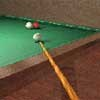#### You may also like### Rain or Shine

Predict future weather using the probability that tomorrow is wet given today is wet and the probability that tomorrow is wet given that today is dry.### Squash

If the score is 8-8 do I have more chance of winning if the winner is the first to reach 9 points or the first to reach 10 points?### Snooker Frames

It is believed that weaker snooker players have a better chance of winning matches over eleven frames (i.e. first to win 6 frames) than they do over fifteen frames. Is this true?

# Snooker

##### Age 16 to 18Challenge Level

Thank you Sue Liu of Madras College, St Andrews for this solution.

This could be any 'best of 15 games' contest between two players where the object is to be the first to win 8 games (called frames in snooker) and the probability $p$ of winning a single game is constant.

We have to find the probability of player A winning the snooker match by adding the probabilities for all the possible outcomes. Player A can win in 8 frames (by winning the first 8 frames outright), or by winning any 7 of the first 8 then winning the ninth (when the match lasts 9 frames), or by winning any 7 of the first 9 then winning the tenth (when the match last 10 frames), or similarly player A can win a match which lasts for 11, 12 13 14 or 15 frames. Note that the last game, which decides the contest, must be won by A. Let $P(x)$ denote the probability of A winning a match with $x$ games in total.

$$\begin{eqnarray} P(8) &= {7\choose 7}p^7p \\ P(9) &= {8\choose 7}p^7(1-p)p\\ P(10)&= {9\choose 7}p^7(1-p)^2p \\ \ldots \\ P(15)&= {14\choose 7}p^7(1-p)^7p \end{eqnarray}$$

Let $f(p)$ denote the probability of A winning a 'best of 15' match when the probability of winning each frame is $p$. $p = P(8) + P(9) + P(10) + P(11) + P(12) + P(13) + P(14) + P(15).$

When $p = 0.4$ this gives the probability $f(0.4)$ of A winning a 'best of 15' match to be 0.2131.

When the probability of A winning each frame is $p = 0.55$ then the probability of the opponent winning a frame is $1-p = 0.45$ and the probability $f(0.55)$ of A winning the match is 0.6535.

When the probability of A winning each frame is $p = 0.5$ then the probability of A winning the match is 0.5 as expected.